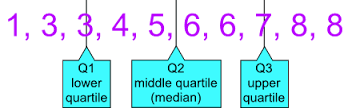Scroll

# Any more tips on GRE percentiles, quartiles, interquartile ranges, and statistics?

When you see a question relating to percentiles, quartiles/interquartile ranges, or boxplots, imagine that you take all your data points and line them up from smallest (on the left) to largest (on the right). Find the middle point, which has an equal number of data points on either side. This is your 50th percentile, also known as the median or middle quartile. Next, find the middle point in the bottom half of the data set: this is your 25th percentile (lower quartile). Finally, find the middle point of the upper half of the data set: this is your 75th percentile (upper quartile). We can see this illustrated in the following picture:The key is to remember that the percentile/quartile refers to how many data points fall above, below, or between certain points. Each quartile contains 25% of the points in the data set. The median is the point with 50% of the points below it and 50% of the points above it. The interquartile range includes the middle 50% of the data points.

In the example above, we have 10 numbers in our set. This means that the 90th percentile has 90%  (9/10) of the data below it and 10% (1/10) above it: this is given by the first 8. The 40th percentile is marked by the number 4 in this data set because 40% (4/10) of the data points are below it and 60% (6/10) of the data points are above it.

The interquartile range is the range between the lower and upper quartile. This is given by the upper quartile value minus the lower quartile value. In the data set above, this is 7-3=4.